MathMonday, January 6no studentsTuesday, January 7 Review Equations and Distributive Property 3 That Quiz assignments Wednesday, January 8Review Topic 1 and 2Thursday, January 9Review Topic 3Friday, January 10Topic 4.5***Extra Videos  **** If you need extra help click on these links to watch videos that help with comprehension.  *****Adding Fractions Video https://www.youtube.com/watch?v=N-Y0Kvcnw8gAdding Mixed Numbershttps://www.youtube.com/watch?v=pynfj2bYRmsMultiplying Fractionshttps://www.youtube.com/watch?v=qmfXyR7Z6LkSimplifying Fractionshttps://www.youtube.com/watch?v=AtBUQH8TkqcDividing Fractionshttps://www.youtube.com/watch?v=4lkq3DgvmJo-2.1 GCF https://www.youtube.com/watch?v=kyzcHkFMinA- 2.1 part II over finding the product using the GCF and the sum of two numbers. https://www.youtube.com/watch?v=KVz-C8lk8u8-LCMhttps://www.youtube.com/watch?v=Z6-LksV08qUhttps://www.bing.com/videos/search?q=LCM+lets+do+math&&view=detail&mid=EFF95F8AF08564DB7899EFF95F8AF08564DB7899&&FORM=VDRVRV      Important DatesAugust 6--First full daySeptember 2--Labor Day/No SchoolOctober 5--End of 1st 9 wks grading periodOctober 14-18--Fall BreakNovember 11--Veteran's Day observed/No SchoolNovember 27-29--Thanksgiving Break/No SchoolDecember 15--End of 2nd 9 wks grading periodDecember 23-January 3rd--Christmas BreakJanuary 6--No StudentsJanuary 20--MLK Day/No SchoolFebruary 17--President's Day/No SchoolMarch 3--Election Day/No SchoolMarch 7--End of 3rd 9 wks grading periodMarch 16-20--Spring BreakApril 10--Good Friday/No SchoolMay 21--Last full day of school VocabularyTo begin, knowing the parts of an expression is very heavy in knowing the vocabulary.  Here is a list of the necessary vocab words with definitions.addend – the numbers added in an addition problem’ 5 + 6 = 11sum – the answer in an addition problem; 5 + 6 = 11difference – the answer to a subtraction problem; 5 – 3 = 2dividend – the first number in a division problem; 6 ÷ 3 = 2divisor – the second number in a division problem; 6 ÷ 3 = 2quotient – the answer in a division problem; 6 ÷ 3 = 2factor – numbers multiplied together to get a product; factors might also be referred to as nunbers that will even go into another; 3 x 4 = 12; 3 and 4 both evenly go into 12, therefore, they are factors of 12product – the answer in a multiplication problem; 3 x 4 = 12monomial – a number, a variable, or a product of numbers or variables; a, 3, 5b, 7a²polynomial – a group of monomials added together; 7a² + 3b + 6;term – each of the monomials within a polynomial;  7a² + 3b + 6; Note: When referencing terms, subtraction is thought of as adding the negative, so if our polynomial were 6a – 3b, then the two terms would be 6a and -3b.coefficient – the number a variable is being multiplied by; 3a, 4b; One thing to note with this is if a variable does not have a number multiplying it, like ‘c’, then it’s coefficient is 1 because there is only one c there.——————————————————————————There are also less commonly used words, but might be fun to know:augend – the proper name for the first number in an addition problem; 3 + 4 = 7minuen – the first number in a subtraction problem; 5 – 2 = 3subtrahend – the second number in a subtraction problem; 5 - 2 = 3multiplicand – the first number in a multiplication problem; 4 x 5 = 20multiplier – the second number in a multiplication problem; 4 x 5 = 20——————————————————————————With these terms, the main goal is to be able to identify all of the separate parts within an expression.  This skill will be checked in several ways.  Here are some examples:***How many terms are in the following expression?7km + 4 + 7k + 8n + 8n³pThe hard part for the students is to look past the difficulty of the expression and simply answer the question.  In that expression there are 5 terms, each of them separated by the addition sign.***Name the terms in the following expression.4qr – 8Remembering that subtraction is considered addition of a negative, the terms in this expression are 4qr and -8.***In the expression 12 + 46a³, what is the coefficient of a³?Remember, you are looking for the number that the a³ is being multiplied by, so the answer to this question would be 46.Variables and ExpressionsThis lesson has to do with the taking expressions from words into number form.  A lot of the vocabulary from the previous lesson comes into play here because it requires knowing what they mean.  Examples: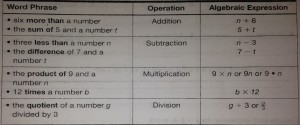Another aspect of this lesson, which we have already begun to work on as separate tasks, is taking a number and putting it in the place of a variable.Example:Evaluate the expression 9n for n = 3, 5, and 7.To solve this, you would simply put the numbers in for the n.  9(3)=27, 9(5)=45, and 9(7)=63.Properties of EqualityThis lesson takes a closer look at that word equal and what it really means.  We can think of an equation much like you would a scale where the middle of the scale is the equal sign.  Let’s start with 1=1.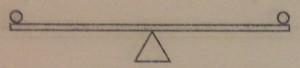Currently the scale is balanced.  We can make changes to that scale as much as we want, so long as we remember that whatever we add or subtract from one side, we have to do the exact same to both.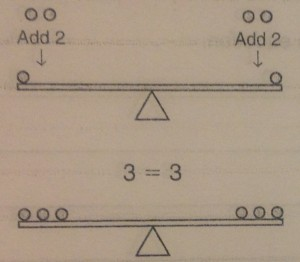The most important part of this is remembering that bit. Whatever you do to one side, has to be done to the other, otherwise the expression is no longer equal.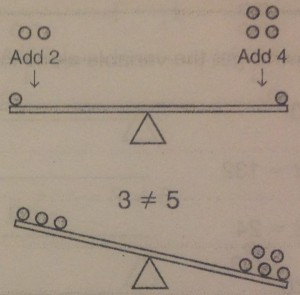We use these properties of equality as we start moving into algebra as it is the key to keeping equations equal while solving for the variable.  Let’s say we have the problem x+5=21, and we have been asked to figure out what x equals.  To do this, we need to get the variable alone.  We could think of this on the same scale.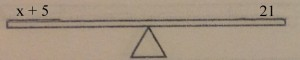To get the variable alone, we need to get rid of the +5.  The two key words are “opposite operation”.  To get rid of a term like that we need to put it’s opposite on the scale.  The opposite of +5 is -5.  Remember though, whatever we do to one side, we have to do to both sides.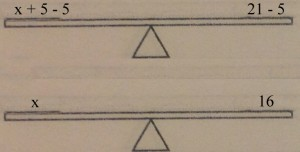We’ve decided that x=16.  We could then go back and check our answer by putting 16 in for the x in the original equation.  16+5=21 is true, so our answer is correct.  These same rules apply for all operations, so knowing the opposites is one of the most important parts of this lesson.  The opposite operations are addition and subtraction, and multiplication and division.Combining Like TermsCombining Like Terms is a process used to simplify an expression or an equation using addition and subtraction of the coefficients of terms. Consider the expression 5 + 7. By adding 5 and 7, you can easily find that the expression is equivalent to 12The key to understanding Combining Like Terms is the meaning of the phrase like termsand being able to identify them. Here are a few examples of like terms:***The following terms are like because they have just one variable, x, with a coefficient:2x, 45x, x, -26x, -2x***The following terms are like because they are all just simple numbers:15, -2, 27, 2046, 0.6***The following terms are like because they are y² with a coefficient:3y², y², -y², 26y²Please note that terms with exponents are not like terms without, so 5x² is not a like term to 3x.Algebraic expressions can also be simplified by combining like terms.  consider the equation12x + 7 + 5xBecause 12x and 5x have the same single variable with only a coefficient, they are like terms and can be added together.  12+5=17 so 12x+5x=17x.  The expression would be simplified to17x+7Here is a more complex example:5x2 + 7x + 2 – 2x2 + 7 + xThe commutative property tells us we can rearrange the addition² without consequence. This can help us to put like terms together.(5x² - 2x²) + (7x + x) + (2 + 7)We can then simplify it by doing the basic operations between the like terms.3x² + 8x + 9The following topics will be the areas of focus in sixth grade mathematics:      **The Number System (multiplying and dividing fractions, fluent computation with multi-digit numbers, finding common factors and multiples, applying and extending previous understandings of numbers to the system of rational numbers)     ** Ratios and Unit Rates (understand ratio concepts, use ratio reasoning to solve problems)     **Expressions and Equations (applying and extending previous understandings of arithmetic to algebraic expressions, one-variable equations and inequalities)    **Geometry (area, surface area and volume)     **Statistics and Probability (statistical variability and distributions)Math Notebook Students will be expected to keep a three-ring binder or folder dedicated to math. It is important that you bring your math binder/folder to class everyday as well as to keep it UP-TO-DATE! There is a high correlation between students who are organized and keep good notes to academic success and achievement. Notebooks will be collected and graded.Week of 17th - 21stMonday - Decimals in Arithmetic - review with https://www.mathantics.com/section/lesson-video/decimal-arithmetic  IXL G.1, H.2, H.4 (10 questions in each section during class) This is only homework if not completed during school hours.  Tuesday - Finish IXL G.1, H.2, H.4 (first 10 on Monday)Wednesday - Review Decimals - Notes in Portfolio, Review Decimal Division including a decimal in the divisor and dividend.   Monday - September 10th - Fraction Division Quiz and Lesson over fraction word problems.https://www.youtube.com/watch?annotation_id=annotation_962858231&feature=iv&src_vid=LMeGiCov6vo&v=f4-xdO3vQOgTuesday - September 11th - Module 5 Dividing https://my.hrw.com/content/hmof/math/gomath/na/gr6/online_interactive_teacher_book_9780544518971_/G6_U2_M5_L1/launch.htmlWednesday - September 12th- IXL C.5 and C.6Thursday- September 13th - IXL F.1 and F.2Friday, September 14th - In class we completed a "That Quiz" and reviewed multi-digit division.  Homework - Watch the Math Antic Video "Decimal Arithmetic" in the 1st tab, scroll down to the middle of the list. https://www.mathantics.com/section/lesson-video/decimal-arithmeticThursday, September 6th - That Quiz - If you didn't finish in class then complete up to 5  problems as homework.  Here are two videos over dividing fractions and dividing mixed numbers:https://www.mathantics.com/section/lesson-video/intro-to-mixed-numbersFriday, September 7th - That Quiz  review/practice over Dividing Mixed Numbers and inequalities https://www.mathantics.com/section/lesson-video/dividing-fractions August 2018Monday, August 20th - Lesson 3.1 Classifying Rational NumbersTuesday, August 21st - Identifying Opposites and Absolute Value of Rational NumbersWednesday, August 23rd - Comparing and Ordering Rational NumbersThursday, August 24th - 3.3 Ordering Rational Numbers Friday - Study Guide ReviewMonday, August 13th - LCM (Least Common Multiple) and GCF (Greatest Common Factor) worksheet in class. Remember Test Tomorrow!Tuesday - Test over GCF and LCMWednesday - positive and negative integersThursday - Absolute Value and inequalitiesMonday, August 6th - Dividing Fractionshttp://www.mathantics.com/section/lesson-video/dividing-fractionsTuesday - Beginning of the year diagnostic testWednesday - Greatest Common Factorhttps://www.shmoop.com/video/lcm-gcfThursday - review GCF and complete Lesson 2-1 pages 20,22-24 in class.  Friday - Least Common Multiple (LCM)Links:Math Gameshttps://www.mathgames.com/grade6